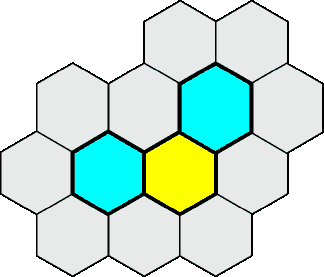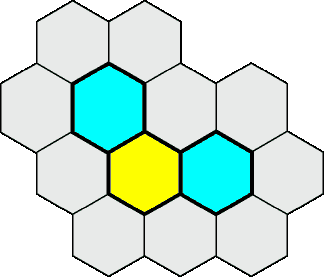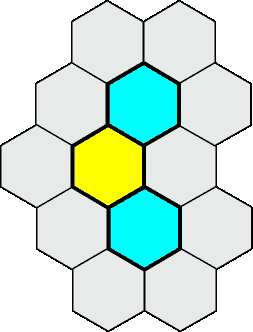# Hex Cellular Automaton

This is a totalistic cellular automaton on a hexagonal field with three colors. Totalistic means, that for calculating the next color, only the number of colors on the six neighbour fields are counted and the position is not considered. The ruleset for calculating the new color (result) is very easy:

```byte result = center;
if (s1 == 0 && s2 == 1 && center == 0) {
result = 2;
} else if (s1 == 1 && s2 == 1) {
result = 1;
} else if (s2 == 2) {
result = 1;
} else if (s2 == 3) {
result = 2;
} else {
result = 0;
}
```

s0, s1 and s2 are the number of color 0, 1 and 2 on the six neighbour fields. s0 is not used in the rule set.

Try to click and drag in the Applet area! Left mouse button changes from empty to cyan to yellow and right mouse button changes back from yellow to cyan to empty.

There are some interesting patterns. For example a spinning emitter:This emitter produces six different gliders while spinning:The source: HexAutomaton.java and HexAutomatonCanvas.java20. Januar 2003, Frank Buß